Reach Us+44-7480-724769
Verification of Some Properties of the C-nilpotent Multiplier in Lie Algebras | OMICS International
Journal of Generalized Lie Theory and Applications
All submissions of the EM system will be redirected to Online Manuscript Submission System. Authors are requested to submit articles directly to Online Manuscript Submission System of respective journal.

Verification of Some Properties of the C-nilpotent Multiplier in Lie Algebras

Yazd Branch, Islamic Azad University, Iran

Corresponding Author:
Yazd Branch, Islamic Azad University, Iran
Tel: +982147911
E-mail: [email protected]

Received date: April 21, 2015 Accepted date: July 20, 2015 Published date: July 29, 2015

Citation: Sadeghieh A, Araskhan M (2015) Verification of Some Properties of the C-nilpotent Multiplier in Lie Algebras. J Generalized Lie Theory Appl 9:228. doi:10.4172/1736-4337.1000228

Copyright: © 2015 Sadeghieh A, et al. This is an open-access article distributed under the terms of the Creative Commons Attribution License, which permits unrestricted use, distribution, and reproduction in any medium, provided the original author and source are credited.

Visit for more related articles at Journal of Generalized Lie Theory and Applications

Abstract

The purpose of this paper is to obtain some inequalities and certain bounds for the dimension of the c-nilpotent multiplier of finite dimensional nilpotent Lie algebras and their factor Lie algebras. Also, we give an inequality for the dimension of the c-nilpotent multiplier of L connected with dimension of the Lie algebras γd (L) and L / Zd−1 (L) . Finally, we compare our results with the previously known result.

Keywords

C-nilpotent multiplier; Nilpotent lie algebra; Lie algebra

Introduction

All Lie algebras referred to in this article are (of finite or infinite dimension) over a fixed field F and the square brackets [ , ] denotes the Lie product. Let 0→R→F→L→0 be a free presentation of a Lie algebra L, where F is a free Lie algebra. Then we define the, c-nilpotent multiplier c ≥ 1, to be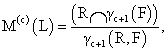where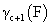is the (c+1)-th term of the lower central series of F, γ1(R, F) = 1 and γc+1(R,F) = [γc(R,F),F]. This is analogous to the definition of the Baer-invariant of a group with respect to the variety of nilpotent groups of class at most c given by Baer [1-3] (for more information on the Baer invariant of groups). The Lie algebra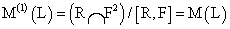is the most studied Schur multiplier of L [4,5]. It is readily verified that the Lie algebra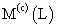is abelian and independent of the choice of the free presentation of L . The purpose of this paper is to obtain some inequalities for the dimension of the c-nilpotent multiplier of finite dimensional nilpotent Lie algebras and their factor Lie algebras (Corollary 2.3 and Corollary 2.5). Finally, we compare our results to upper bound given . First, we show that for each ideal N in L, there is a close relationship between the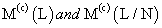Lemma 1.1. Let L be Lie algebra with a free presentation 0→R→F→L→0. If S is an ideal in F with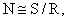, then the following sequences are exact: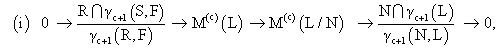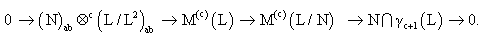under the condition that N is central,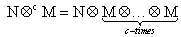and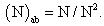Proof. We prove only part (ii). Since N is central,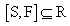and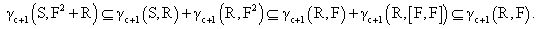Now, we have the following homomorphism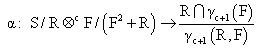such that Imα=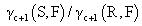. Now the result holds by part (i).

The following corollary is an immediate consequence of Lemma 1.1, which gives some elementary results about dimension of the c-nilpotent multiplier of finite dimensional Lie algebras see corollary 2.2 of Salemkar et al. .

Corollary 1.2. Let N be an ideal of Lie algebra L. Then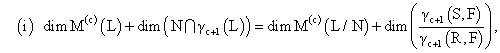Where F,S,R are defined in Lemma 1.1.

(ii)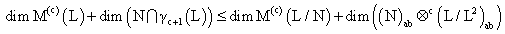Suppose that L is generated by n elements. Let F be a free Lie algebra generated by n elements and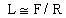Witt,s formula from Bahturin et al.  gives us

Dim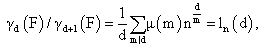where μ(m) is the Mobius function, defined by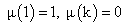if K is divisible by a square, and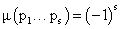if p1,….,ps are distinct prime numbers.

Lemma 1.3. Let L be an abelian Lie algebra of dimension n. Then

dim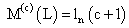. In particular, dim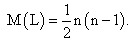Proof. Consider a free Lie algebra F freely generated by n elements. By Witt’s formula, F/F2 is an abelian Lie algebra of dimension n, and so it is isomorphic to L. Hence dim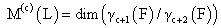, which gives the result.

Let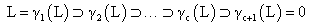be the lower central series of nilpotent Lie algebra, L. L is said to have class c if c is the least integer for which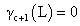. Furthermore, if dim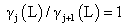for j=2,3,…,c and dim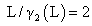, then L is said to be of maximal class c. Additionally, let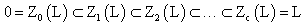be the upper central series of nilpotent Lie algebra L. If L is of maximal class, then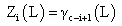for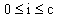.

By the above notation we have the following corollary.

Corollary 1.4. Let L be a finite dimensional nilpotent Lie algebra of maximal class (c+1), then

dim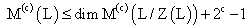Proof. Using Corollary 1.2(ii) with N= Z(L), we get

dim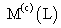+dim Z(L) = dim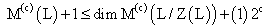Discussion and Results

2 Bounds on dimLet L be a finite dimensional nilpotent Lie algebra of class d > 2. First, we give an inequality for the dimension of the c-nilpotent multiplier of L connected with dimension of the Lie algebras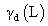and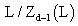(Corollary 2.3) and some inequalities for the dimension of the c-nilpotent multiplier of finite dimensional nilpotent Lie algebras will be given. For this purpose, we need the following two lemmas.

Lemma 2.1. Let H and N be ideals of Lie algebra L and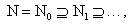a chain of ideals of N such that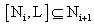for all I = 1,2,…. Then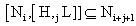for all i, j.

Proof. We have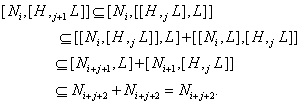Now, the assertion follows by induction on j.

Lemma 2.2. Let L be a finite dimensional nilpotent Lie algebra of class d ≥ 2 . Let 0→R→F→L→0 be a free presentation of L, then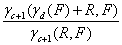is a homomorphic image of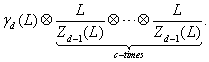Proof. Put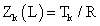for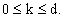. Now consider the following chain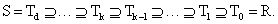Since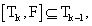then by Lemma 2.1,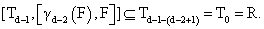Therefore,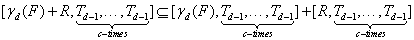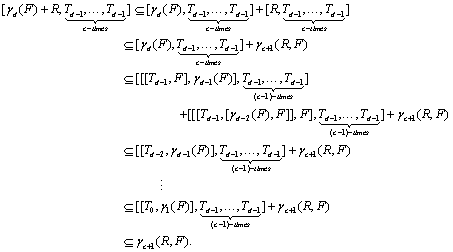The latter inclusion gives the following epimorphism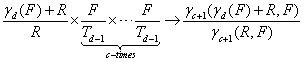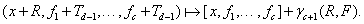Corollary 2.3. Under the assumptions and notation of the above Lemma, we have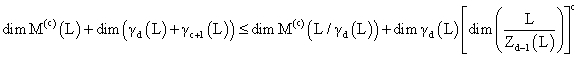Proof. In Corollary 1.2(i), taking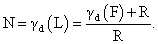Now by Lemma 2.2, we have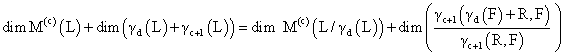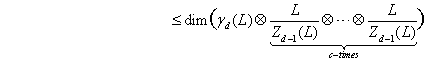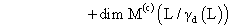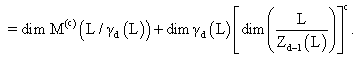In following, we give another an inequality for the dimension of the c-nilpotent multiplier of finite dimensional nilpotent Lie algebras.

Theorem 2.4. Let L be a finite dimensional nilpotent Lie algebra of class ≥ 1 , then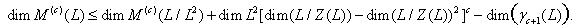Proof. We use induction on the class of L. If L is of class 1, then L2 = 0 and the result holds. Assume the result for nilpotent Lie algebras of class to be less than d and let L have class m= d-1. Note that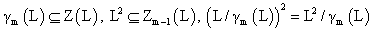and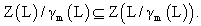For convenience, let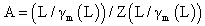and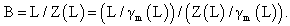Since A is a homomorphic image of B, it follows that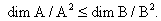By induction,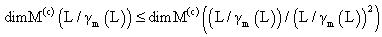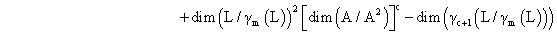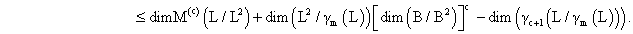By Corollary 2.3,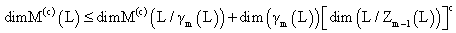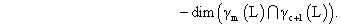Also,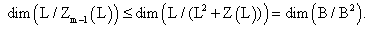Therefore,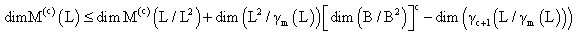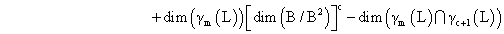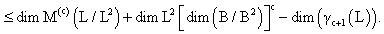Since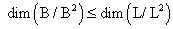, we obtain:

Corollary 2.5. Under the assumptions and notation of the above Theorem, we have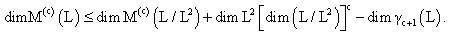Now, we compare our results to upper bound given , when c = 1.

Theorem 2.6. Let L be a finite dimensional nilpotent Lie algebra of class m and d=d(m). Then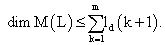Example 2.7. Let F be a free Lie algebra on 2 generators and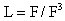. Then L is a Lie algebra of 2 generators and class 2. Thus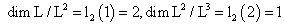and dim L=3. By Theorem 2.6,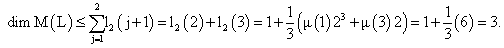Note that L is a finite dimensional nilpotent Lie algebra of maximal class (1 + 1) and Z(L)=L2. By Corollary 1.4 and Lemma 1.3,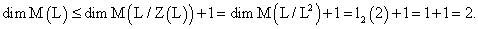Also, by Corollary 2.5,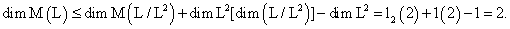Example 2.8. Let F be a free Lie algebra on 2 generators and L = F / F4 . Then L is a Lie algebra of 2 generators and class 3. Thus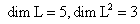and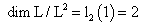. By Theorem 2.6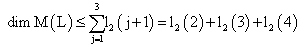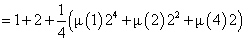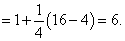Also, by Corollary 2.5,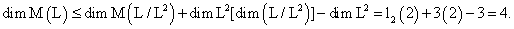In this two examples, we see that our results give two better upper bounds for dim(L) than the previously known result.

Acknowledgements

The authors wish to thank Yazd Branch, Islamic Azad University for its support of research project under the title Verification of some properties of The c-nilpotent multiplier in Lie algebras.

References

Select your language of interest to view the total content in your interested language

Article Usage

• Total views: 12671
• [From(publication date):
December-2015 - Oct 23, 2019]
• Breakdown by view type
• HTML page views : 8680Can't read the image? click here to refresh